# Im>0?

Is -10i a positive number?

Result

#### Solution:

No, complex (imaginary) numbers are not ordered and therefore does not make sense to compare them.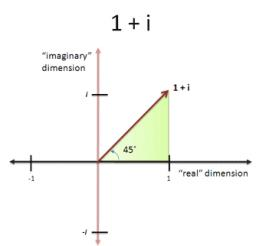Our examples were largely sent or created by pupils and students themselves. Therefore, we would be pleased if you could send us any errors you found, spelling mistakes, or rephasing the example. Thank you!

Leave us a comment of this math problem and its solution (i.e. if it is still somewhat unclear...):Be the first to comment!Tips to related online calculators

#### You need to know the following knowledge to solve this word math problem:

We encourage you to watch this tutorial video on this math problem:

## Next similar math problems:

1. The modulus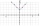Find the modulus of the complex number 2 + 5i
2. Imaginary numbersFind two imaginary numbers whose sum is a real number. How are the two imaginary numbers related? What is its sum?
3. Goniometric form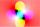Determine goniometric form of a complex number ?.
4. Complex number coordinatesWhich coordinates show the location of -2+3i
5. Linear imaginary equationGiven that ? "this is z star" Find the value of the complex number z.
6. ABS CNCalculate the absolute value of complex number -15-29i.
7. BearingA plane flew 50 km on a bearing 63°20' and the flew on a bearing 153°20' for 140km. Find the distance between the starting point and the ending point.
8. Moivre 2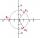Find the cube roots of 125(cos 288° + i sin 288°).
9. De Moivre's formula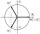There are two distinct complex numbers z such that z3 is equal to 1 and z is not equal 1. Calculate the sum of these two numbers.
10. Log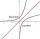Calculate value of expression log |3 +7i +5i2| .
11. ReciprocalCalculate reciprocal of z=0.8-1.8i:A domed stadium is in the shape of spherical segment with a base radius of 150 m. The dome must contain a volume of 3500000 m³. Determine the height of the dome at its centre to the nearest tenth of a meter.Are these numbers 2i, 4i, 2i + 1, 8i, 2i + 3, 4 + 7i, 8i, 8i + 4, 5i, 6i, 3i complex?